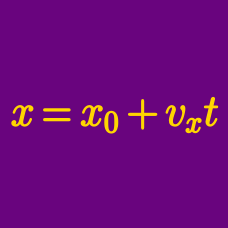Classical Mechanics

# Position-time graph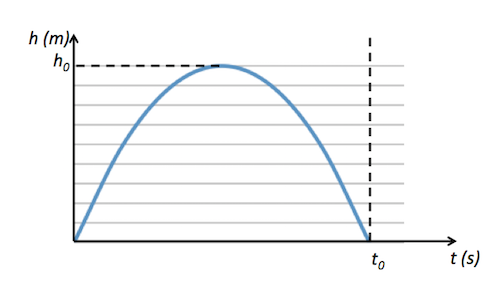A $300 \text{ g}$ football is kicked in a direction that makes a $30^\circ$ angle with the horizon. The above graph depicts the vertical position (in meters) of the football. If $h_0 =80,$ how long (in seconds) does the football fly before falling back to the ground?

The air resistance is negligible and the gravitational acceleration is $g = 10 \text{ m/s}^2.$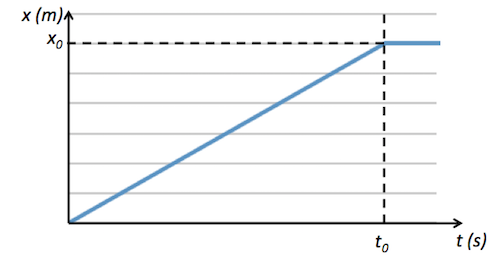The above graph depicts the horizontal position of a ball that is thrown horizontally at $23 \text{ m/s} .$ If $x_0=69 \text{ m},$ from what height was the ball thrown?

The gravitational acceleration is $g= 10 \text{ m/s}^2.$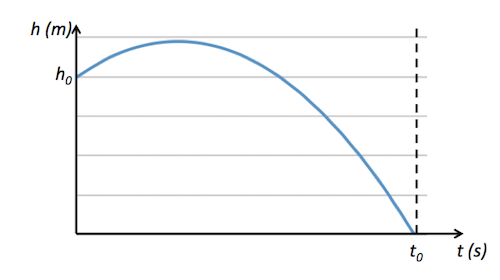A body of mass $m = 3 \text{ kg}$ is projected in a direction that makes a $\theta = 30^\circ$ angle with the horizon. The above graph shows the vertical position of the body with respect to time $t$ (in seconds). If $t_0= 8 \text{ s}$ and $h_0=160 \text{ m},$ how far does the body fly horizontally?

The air resistance is negligible and the gravitational acceleration is $g = 10 \text{ m/s}^2.$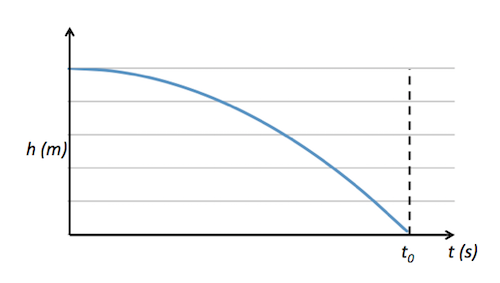A body of mass $m = 1 \text{ kg}$ is projected horizontally with a velocity of $v= 6 \text{ m/s}.$ The above graph shows the vertical position of the body with respect to time $t$ (in seconds). If $t_0= 8 \text{ s} ,$ from what height was the body projected?

The air resistance is negligible and the gravitational acceleration is $g = 10 \text{ m/s}^2.$A $9\text{ kg}$ ball is projected from the ground in a direction that makes a $30 ^\circ$ angle with the horizon. The above graph depicts the vertical position of the ball during its flight. If $t_0=8\text{ s}$ and $h_0 =80\text{ m},$ how far does the ball fly horizontally?

The air resistance is negligible and the gravitational acceleration is $g = 10 \text{ m/s}^2.$

×